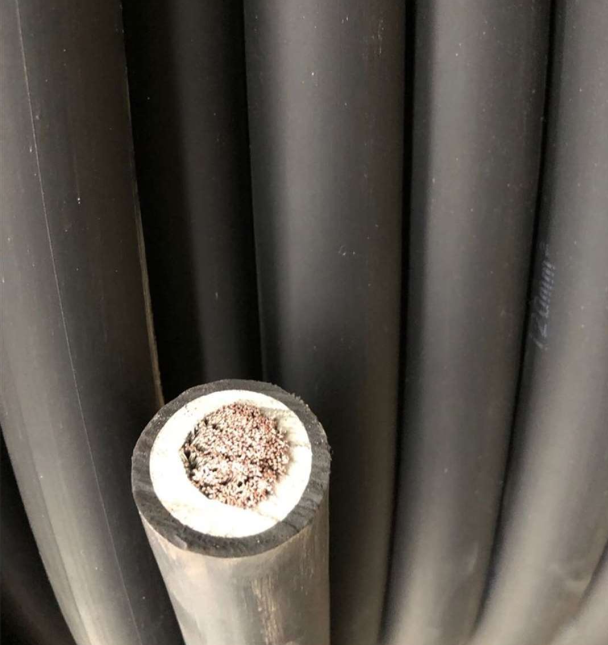13637026879

电线电缆成缆计算公式

1

3+1（主线为扇形），其成缆外径近似公式D=2.31h

4芯（为扇形），其成缆外径近似公式D=2.2h

3芯（为扇形），其成缆外径近似公式D=2.11h

3+24+1（主线为瓦形），其成缆外径近似公式D=2h+d（园形线芯外径） 三大一小圆形：D=(3*d+d)*0.61

2

2等芯 D=2d ②3等芯 D=2.16d

4等芯 D=2.42d ④5等芯 D=2.7d

67）等芯 D=3d ⑥3*1 D=2.4(3d+d)/4

3+2 D=2.7(3d+2d)/5 ⑧4+1 D=2.74d+d小）/5

2等芯 D=2d ②3等芯 D=2.16d

3+1 D=2.31(3d+d)/4 ④4等芯 D=2.31d

5等芯 D=2.42d ⑥4+1 D=2.424d+d小）/5

3+2 D=2.423d+2d小）/5• 打印本文
• 关闭本页
• 留言评论
• 推荐度：
•